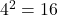# 4-Variable K-Map

Previous post, you learned about 3-variable K-map, and learned how to minimize a boolean function. In this post, you will learn about bigger map such as a 4-variable K-map. With 4-variable map you will be able to make larger groups of cells.

## Plotting a 4-variable K-map

The 4 variables of a Boolean function will give a truth table of aboutrows of input combinations. These can be directly translated to 16 cell 4-variable K-map. See the following figure.

## Grouping of cells in a 4-variable K-map

While you minimize a boolean function using 4-variable K-map, group the cell with 1s into 2s, 4s, and 8s, and so on. See the figure below.

## Rules for Grouping of cells

Here are some simple rules when group cells.

• Single cells with 1 gives you a term with 4 literals.
• Two adjacent cells will minimize and give you a term with 3 literals.
• A grouping of 4 cells of 1s will give you a term with 2 literals.
• A grouping of 8 cells of 1s will give a single literal.

Therefore, you must always try for maximum number of 1s in a group. Some group overlap each other as we mentioned in earlier in previous post. Each overlapping group must include one uncovered cell that contains a 1. See the figure below to understand this.

## 4-Variable K-Map Example

In this section, I have given few examples of 4-variable K-maps. For more practice, you can refer to some textbooks with lot of exercises.

### Q1: Minimize the following Boolean function using 4-variable K-map.

F(A,B,C,D) = ∑(0,1,2,4,5,6,8,9,12,13)

Solution:

Step 1: Construct a 4-variable K-map and mark all minterms with 1.

Step 2: Look vertically in a selected group and extract any common variable. Also look horizontally, extract any common variable from the group.

In the group of four, horizontally, you get D’ and vertically, you get A’. Together it is a term of two literal, that is, A’D’. Similarly, the group of 8, vertically everything cancels out and leave just 1 because A’B’ + A’B = A’ and AB + AB’ = A, finally, A’ + A = (1). But, horizontally, for the group of 8, you get C’. Always the group of 8 will give you single literal.

Step 3: Write down all the solution.

Group of 8 = C'
Group of 4 = A'D'
The final expression is F = A'D' + C'

## Verify the Solution using Algebraic Method

Let’s verify our solution using Boolean algebraic method.

F(A,B,C,D) = ∑(0,1,2,4,5,6,8,9,12,13)
F = A'B'C'D' + A'B'C'D + A'B'CD' + A'BC'D'+ A'BC'D + A'BCD' + AB'C'D' + AB'C'D + ABC'D' + ABC'D
F = A'B'C'(D' + D) + A'CD'(B' + B) + A'BC'(D' + D) + AB'C'(D'+ D) + ABC'(D' + D)
F = A'B'C' + A'CD' + A'BC' + AB'C' + ABC'
F = A'C'(B' + B) + AC'(B' + B) + A'CD'
F = A'C' + AC' + A'CD'
F = C'(A' + A) + A'CD'
F = C' + A'D' // variable C has no effect hence removed

From the solution above it is clear that the 4-variable K-map is a simple solution to minimize a Boolean function with 4 variables.